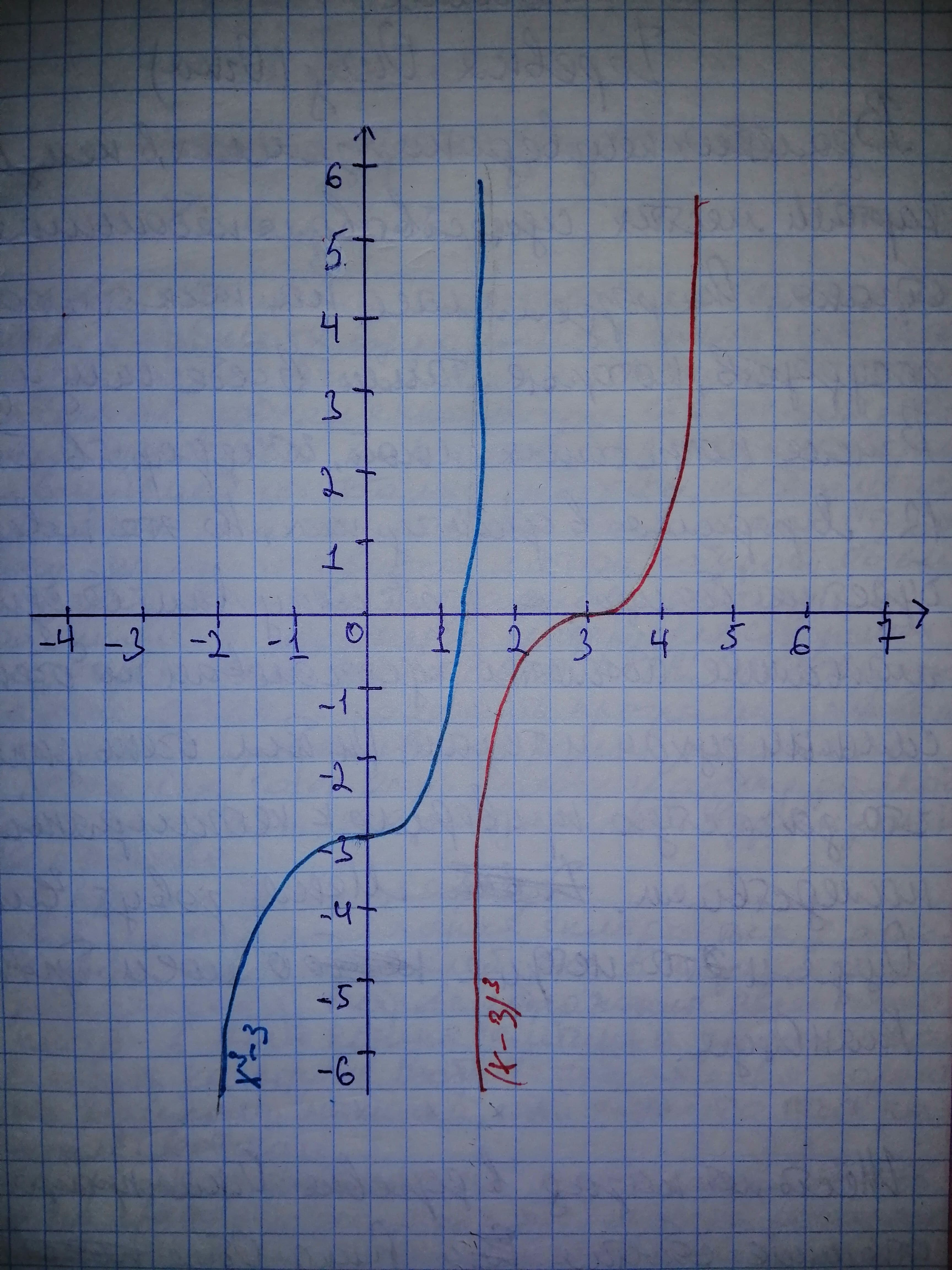Question# Let f(x)=x^{3} and g(x)=x - 3. a) Determine the equations of the composite functions (f circ g)(x) and (g circ f)(x). b) Graph the composite functions. c) Graph the composite functions. Describe (f circ g)(x) and (g circ f)(x) as transformations of f(x).

Transformations of functions
ANSWEREDLet $$\displaystyle{f{{\left({x}\right)}}}={x}^{{{3}}}\ {\quad\text{and}\quad}\ {g{{\left({x}\right)}}}={x}\ -\ {3}.$$ a) Determine the equations of the composite functions $$\displaystyle{\left({f}\ \circ\ {g}\right)}{\left({x}\right)}\ {\quad\text{and}\quad}\ {\left({g}\ \circ\ {f}\right)}{\left({x}\right)}.$$ b) Graph the composite functions. c) Graph the composite functions. Describe $$\displaystyle{\left({f}\ \circ\ {g}\right)}{\left({x}\right)}\ {\quad\text{and}\quad}\ {\left({g}\ \circ\ {f}\right)}{\left({x}\right)}$$ as transformations of f(x).2020-11-13

Step 1 Given: $$\displaystyle{f{{\left({x}\right)}}}={x}^{{{3}}}$$
$$\displaystyle{g{{\left({x}\right)}}}={x}\ -\ {3}$$ a) Let us use the definition of composite functions $$\displaystyle{\left({f}\ \circ\ {g}\right)}{\left({x}\right)}={f{{\left({g{{\left({x}\right)}}}\right)}}}$$ along with the definitions of f and g to determine the composite functions. $$\displaystyle{\left({f}\ \circ\ {g}\right)}={f{{\left({g{{\left({x}\right)}}}\right)}}}={f{{\left({x}\ -\ {3}\right)}}}={\left({x}\ -\ {3}\right)}^{{{3}}}$$
$$\displaystyle{\left({g}\ \circ\ {f}\right)}{\left({x}\right)}={g{{\left({f{{\left({x}\right)}}}\right)}}}={f{{\left({x}^{{{3}}}\right)}}}={x}^{{{3}}}\ -\ {3}$$

Step 2 b) We need to graph the two functions $$\displaystyle{\left({f}\ \circ\ {g}\right)}{\left({x}\right)}={\left({x}\ -\ {3}\right)}^{{{3}}}\ {\quad\text{and}\quad}\ {\left({g}\ \circ\ {f}\right)}{\left({x}\right)}={x}^{{{3}}}\ -\ {3}.$$ For a $$\displaystyle{T}{I}\frac{{83}}{{84}}-{c}{a}{l}{c}\underline{{a}}\to{r},\ {p}{r}{e}{s}{s}\ \text{Y=},\ {t}{h}{e}{n}\ {e}{n}{t}{e}{r}{\ (X\ -\ 3)^{3}}\ {a}{f}{t}{e}{r}{Y}{1}\ {\quad \ and \ {e}{n}{t}{e}{r}}\ \text{X^{3 - 3}}\ {a}{f}{t}{e}{r}\ {Y}{2}.$$ Next, press GRAPH to graph the two curves.Step 3 c) A function of the form $$\displaystyle{g{{\left({x}\right)}}}={a}\ {f{{\left({b}{\left({x}\ +\ {c}\right)}\right)}}}\ +\ {d}\ \text{is obtained by stretching the function}\ {f{{\left({x}\right)}}}\ \text{vertically by a factor}\ {a}{\left({a}\ {<}\ {0}\ {f}{l}{i}{p}{s}\ {t}{h}{e}\ {f}{u}{n}{c}{t}{i}{o}{n}\ {u}\psi{d}{e}\ {d}{o}{w}{n}\right)},\ \text{stretching the function horizontally by a factor}\ {b}{\left({b}\ {<}{0}\ {f}{l}{i}{p}{s}\ {t}{h}{e}\ {g}{r}{a}{p}{h}\ \le{f}{t}-\right)},\text{moves the function to the left by c units}\ {\left(\to\ {t}{h}{e}\ {r}{i}{g}{h}{t}\ {w}{h}{e}{n}\ {c}\ {<}\ {0}\right)}\ \text{and moves the function up by d units}\ {\left({d}{o}{w}{n}\ {w}{h}{e}{n}\ {d}\ {<}\ {0}\right)}.$$ We note that the function $$\displaystyle{\left({f}\ \circ\ {g}\right)}{\left({x}\right)}={\left({x}\ -\ {3}\right)}^{{{3}}}\ \text{is the function}\ {f{{\left({x}\right)}}}={x}^{{{3}}}$$ moved 3 units to the right. We also note that the function $$\displaystyle{\left({g}\ \circ\ {f}\right)}{\left({x}\right)}={x}^{{{3}}}\ -\ {3}\ \text{is the function}\ {f{{\left({x}\right)}}}={x}^{{{3}}}$$ moved 3 units down.